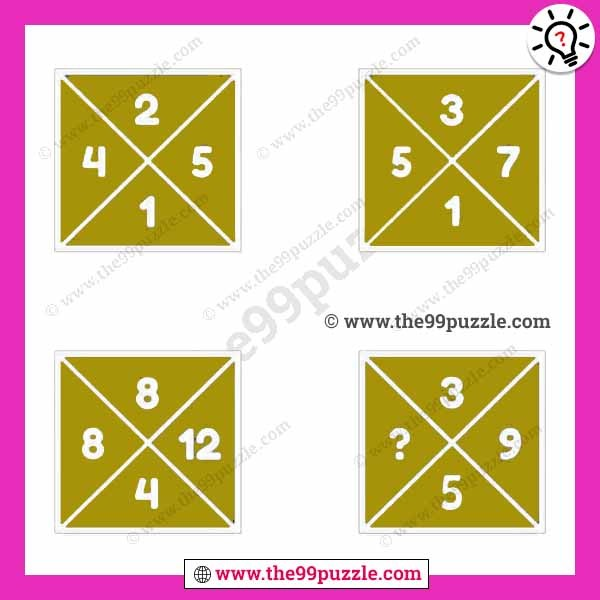# Which number can replace the question mark in this box puzzle? – Puzz135

Which number can replace the question mark in this box puzzle? In this missing number puzzle, we apply a simple logic if you are intelligent you can quickly solve this math problem. Observe this box puzzle carefully and replace the question mark.###### Explanation:

Top Box (Left)= 5−4+1=2

Top Box (Right)= 7−5+1=3

Bottom Box (Left)= 12−8+4=8

Bottom Box (Right)= 9−11+5=3